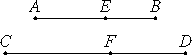# Proposition 70

A straight line commensurable with the side of the sum of two medial areas is the side of the sum of two medial areas.

Let AB be the side of the sum of two medial areas, and CD commensurable with AB.

It is to be proved that CD is also the side of the sum of two medial areas.X.41

Since AB is the side of the sum of two medial areas, divide it into its straight lines at E, therefore AE and EB are straight lines incommensurable in square which make the sum of the squares on them medial, the rectangle contained by them medial, and furthermore the sum of the squares on AE and EB incommensurable with the rectangle AE by EB.

Make the same construction as before.

We can then prove similarly that CF and FD are also incommensurable in square, the sum of the squares on AE and EB is commensurable with the sum of the squares on CF and FD, and the rectangle AE by EB with the rectangle CF by FD, so that the sum of the squares on CF and FD is also medial, the rectangle CF by FD is medial, and moreover the sum of the squares on CF and FD is incommensurable with the rectangle CF by FD.

Therefore CD is the side of the sum of two medial areas.

Therefore, a straight line commensurable with the side of the sum of two medial areas is the side of the sum of two medial areas.

Q.E.D.

## Guide

This proposition is not used in the rest of the Elements